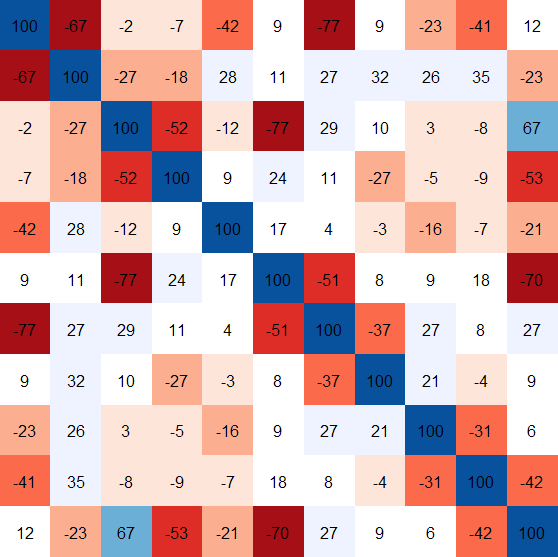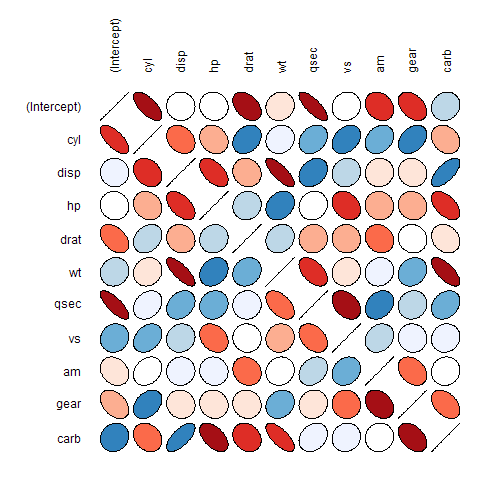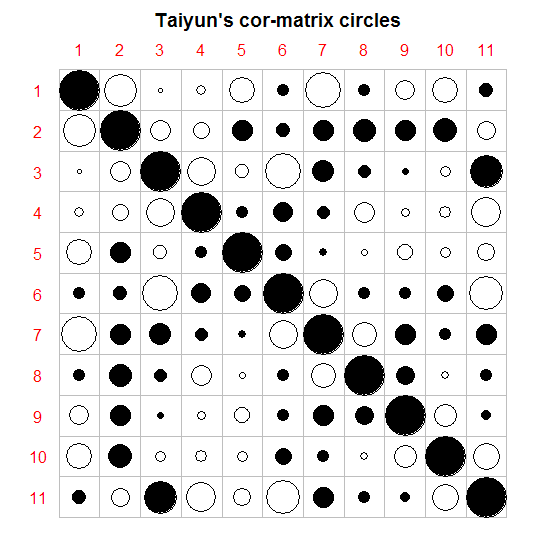2010-4-11更新:本文及扩展工作对应的包corrplot可从CRAN下载。

# 颜色图

R 中，image()函数可以轻松绘制颜色图，利用 mcars 数据，下面代码可以得到其加了相关系数(乘以100，是为了节省空间)的颜色图（图1）。

data(mtcars)
fit = lm(mpg ~ ., mtcars)
cor = summary(fit, correlation = TRUE)\$correlation
#相关阵上下倒转再转置，是为了让画出的图和相关阵方向一致
cor2 = t(cor[11:1, ])
#下面颜色取自ellipse包中的plotcorr函数的示例
colors = c("#A50F15", "#DE2D26", "#FB6A4A", "#FCAE91", "#FEE5D9",
"white", "#EFF3FF", "#BDD7E7", "#6BAED6", "#3182BD", "#08519C")
image(1:11, 1:11, cor2, axes = FALSE, ann = F, col = colors)
text(rep(1:11, 11), rep(1:11, each = 11), round(100 * cor2))# 椭圆图

R 中，ellipse包中的plotcorr() 可以实现这一功能，同样利用 mcars 数据，来展示椭圆图（图2）。他山之石，可以攻玉，椭圆图当然可以利用色彩来增强表现力。

library(ellipse)
col = colors[as.vector(apply(corr, 2, rank))]
plotcorr(cor, col = col, mar = rep(0, 4))# 圆圈图

1. 用圆的面积来表示相关矩阵的绝对值大小。 1.用实心圆(圆内填充颜色)和空心圆来表示相关系数的正负号。

circle.cor=function(cor,axes=FALSE, xlab='', ylab='', asp=1,
title="Taiyun's cor-matrix circles",...){
n=nrow(cor)
cor=t(cor[n:1,]) ##先上下倒转，再转置
par(mar = c(0, 0, 2, 0), bg = "white")
plot(c(0,n+0.8),c(0,n+0.8),axes=axes, xlab='', ylab='', asp=1, type='n')
segments(rep(0.5,n+1),0.5+0:n, rep(n+0.5,n+1),0.5+0:n,col='gray')
segments(0.5+0:n, rep(0.5,n+1), 0.5+0:n, rep(n+0.5,n),col='gray')
for(i in 1:n){
for(j in 1:n){
c=cor[i, j]
bg=switch(as.integer(c&gt;0)+1,'white','black')
symbols(i,j,circles=sqrt(abs(c))/2, add=TRUE, inches=F, bg=bg)
}
}
text(rep(0,n),1:n,n:1,col='red')
text(1:n,rep(n+1),1:n,col='red')
title(title)
}
circle.cor(cor)# 讨论

1. 人们往往更为关注相关性较强的数据，从这一方面来看，椭圆图比较失败，因为它将最大的面积留给了相关性最弱的数据，给其他信息的获取造成了一定的干扰。而颜色图和圆圈图则较为成功，尤其是圆圈图。
2. 相关系数的正负号非常有价值，在从这方面来看，颜色图和椭圆图都比较失败。所谓“五色令人目盲”，当色彩过于纷呈时，我们往往会眼花缭乱，正负号各仅有其一，而颜色图中的颜色却远非如此(这对于色盲更为不利)。而椭圆图中，反映正负号的信息本来就是两个方向，不如圆圈图那样“黑白分明”，更糟的是这些信息受到了过多的干扰。
3. 综上，圆圈图的优点是：1.黑白分明，正负号一清二楚。2.圆的大小表示相关性强度，具有很好的可比性。3.照顾最广大人群(包括颜色不敏感者以及色盲患者)，方便打印，信息不易失真。4.将表现力的最强图形元素留给最有价值的数据(最大的圆留给相关系数最强的数据)，资源配置良好。5.简单明了，干扰信息最少。